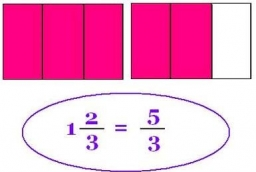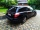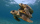# Difference mixed fractions

What is the difference between 4 2/3 and 3 1/6?

d =  1.5

### Step-by-step explanation:Did you find an error or inaccuracy? Feel free to write us. Thank you!Tips to related online calculators
Need help to calculate sum, simplify or multiply fractions? Try our fraction calculator.

## Related math problems and questions:

• Difference of two fractionsWhat is the difference between 1/2 and 1/6? (Write the answer as a fraction in lowest terms. )
• Fractions and mixed numerals(a) Convert the following mixed numbers to improper fractions. i. 3 5/8 ii. 7 7/6 (b) Convert the following improper fraction to mixed number. i. 13/4 ii. 78/5 (c) Simplify these fractions to their lowest terms. i. 36/42 ii. 27/45 2. evaluate following ex
• Mixed numbersRewrite mixed numbers, so the fractions have the same denominator: 5 1/5 - 2 2/3
• Evaluate 17Evaluate 2x+6y when x=- 4/5 and y=1/3. Write your answer as a fraction or mixed number in simplest form.What is 4 1/2+2/7-213/14?
• Evaluate fractionsThe difference  of 5 1/2 and 2/3 is added to the product of 5/6 and 1/2
• RegroupingSubtract mixed number with regrouping: 11 17/20- 6 19/20
• SubtractionTest what do you know about the subtraction of two numbers: a) make a difference if the minuend 4,307,288 and subtrahend is 472008 b) minuend is 4000 more than subtrahend. What's the difference? c) the difference is 38900 less than the minuend. What is th
• Divide 11Divide the product of 4 and 5/8 by 1 1/2. Write your answer as a mixed number.Add two mixed fractions: 2 4/6 + 1 3/6
• A turtleA turtle is 20 5/6 inches below the surface of a pond. It dives to a depth of 32 1/4 inches. What is the change in the turtle’s position? Write your answer as a mixed number.
• Product of the sum and differenceCalculate the product of the sum and difference of numbers -7 and -2.
• Find the 24Find the difference between 2/7 and 1/21Add this two mixed numbers: 1 5/6 + 2 2/11=There are 2 2/3 pizzas. How many people are sharing when each has 2/3 of pizza?The sum of 3 mixed numbers is 20 13/15. two of the numbers are 6 1/3 and 7 5/6. what is the third number?Subtract twice the number -23.6 from the difference of the numbers -130 and -40.2.